Conic Sections
 Home > Lessons > Conic Sections Search | Updated December 25th, 2019
Introduction

In this section, you will learn how to graph conic sections. Here is a list of the sections within this webpage:

 What is a Conic Section? A conic section is a special class of curves. The curves are best illustrated with the use of a plane and a two napped cone. When a plane intersects a two-napped cone, conic sections are formed. The graphic below shows how intersections of a two-napped cone and a plane form a parabola, ellipse, circle, and a hyperbola.[Credit: Pbroks13] How to Graph a Parabola The definition of a parabola is the set of all points that are equidistant to a focal point and a line called a directrix. Howver, it is best recognized by its classic u-shape.      Parabolas either open up, down, right, or left. The graphic below will show you how a parabola looks in comparison to its equation.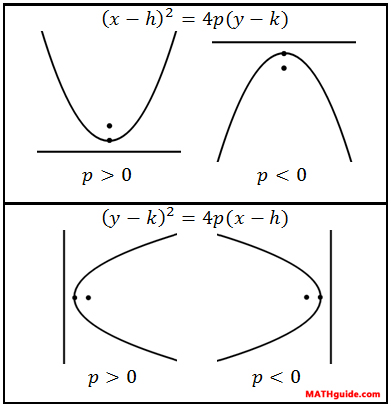When the x-value is being squared, the parabola opens up when the p-value is positive and down when the p-value is negative.      On the other hand, when the y-value is being squared, the parabola opens right when the p-value is positive and left when the p-value is negative.      The h-value and the k-value represents the location of the vertex, V(h,k). Therefore, it is important to know the location of the vertex in order to determine the exact equation of a parabola. Conversely, when the equation of a parabola is known, the exact location of the vertex can be determined.      Here is a video and a quiz that will help you understand and assess your understanding of parabolas.ideo: Parabolas: Directrix, Focus, Vertexideo: Parabolas: Graphingideo: Parabolas: Building Equationsuiz: Parabolas Overview of a Circle The equation of a circle may look intimidating at first glance, but it is simple to use. Here is the equation.The 'h' and the 'k' relate to the center of the circle (h, k) and the 'r' stands for the radius of the circle. The radius is defined as the distance from the center to all points on the circle.      Another definition of a circle is the locus of points in a plane that are all a constant distance, called a radius, from a point, which is called a center. How to Graph a Circle Now that we know what the equation of a circle looks like, let us use an example to see how to graph a specific equation. Here is an equation with specific values.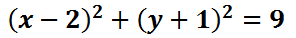To locate the center, take the opposite of the values in the parentheses. The value next to the 'x' is the x-value of the center. The same is true for the value next to the 'y.'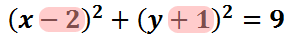So, the center is at (2, -1). We will now graph the center with the circle and explain how it came to be.Now let's turn to the value on the right side of the equation.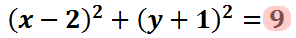The right side of the equation is the square of the radius.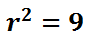To obtain the radius, we take the square root of both sides.This gives us the radius.Now that we have the radius, we can draw the circle. The blue-colored ring is the actual circle. It is the set of points and the points are all three units from its center.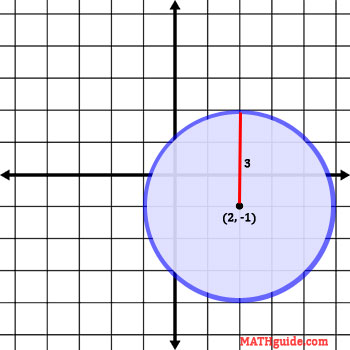Take a look at our quizmaster and activity. They will test your knowledge of equations of a circle to determine if you have digested this information on circles. Use the links that follow.uiz: Equations of Circlesctivity: Equations of Circles
 Overview of an Ellipse An ellipse is defined as a set of points in a plane such that the sum of the distance from two fixed points remains constant. Many people would describe the shape of an ellipse as being oval or close to the outline of an egg shape. This is the equation of an ellipse in standard form.The location of the center of the ellipse is a point located here.The location of the extreme points, called vertices, are located at these points.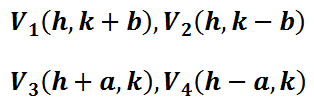The locations of special points called foci are located according to these rules.Foci are important points within an ellipse. Foci are important reflective points that are used by architects for theaters and doctors dealing with kidney stones. Johannes Kepler also described the motion of planets using ellipses for the paths of planets and one focal point for the location of our sun.      Use the lesson below to learn how to graph an ellipse, its center, vertices, and foci. How to Graph an Ellipse Here is the equation of a specific ellipse written in standard form.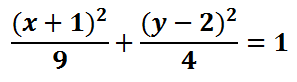In order to graph the ellipse, we need to first locate its center. Its center is obtained by looking at the numbers inside the parentheses.Inside the parentheses with the 'x' there is a 1. Inside the parentheses with the 'y' there is a -2. The center of the ellipse is gained by taking the opposite of these values.The graph below contains the center of the ellipse and the graph of the ellipse. However, we will go through all the steps required to graph the ellipse. The first step is to acquire the center.To proceed, we need to look under the x2-term.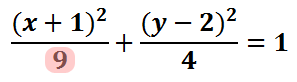This term is the a2 term, if we review the standard form of the ellipse.The value of 'a' is 3; since it was gained from underneath the 'x-value,' we move 3 units left and right from the center to locate two vertices. Here is a picture of those vertices.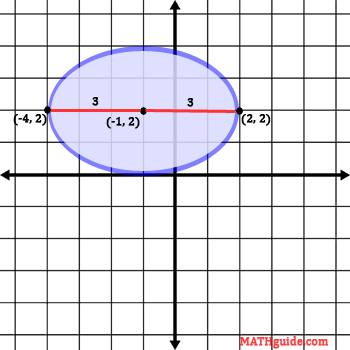To locate the other two vertices, we have to look at the number underneath the y2-term.This term is the b2 term, if we review the standard form of the ellipse.The value 'b' is 2; since it was gained from underneath the 'y-value,' we move 2 units up and down from the center to locate two more vertices. Here is a graph of those vertices.The next steps for graphing this ellipse requires us to know where the major axis is located. The major axis, seen in red below, is the longest distance across the ellipse, which happens to be 6 units in length. The minor axis, seen in yellow below, is the shortest distance across the ellipse, which happens to be 4 units in length.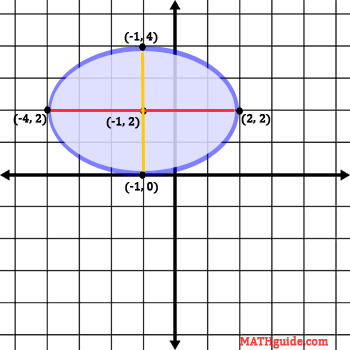The foci are located across on the major axis, seen in red directly above. To locate the foci, we need to look at both values in the denominator from the original equation.The formula for the foci requires us to take the larger of the two values and subtract the smaller, all while underneath a square root.Simplifying further, we get this exact value.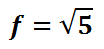The approximation of this value is as follows.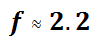We are now going to use this value and move left and right on the major axis to locate the foci.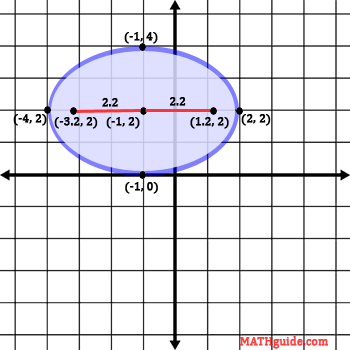Here are the exact calculations for those foci.Here is the final graph of the ellipse with all the special points included.Try our quizmaster and activity on graphing ellipses below to see if you can graph your own ellipse.ideo: Graphing Ellipsesideo: Equations of Ellipses Given Foci and Verticesuiz: Graphing Ellipsesuiz: Equations of Ellipsesctivity: Ellipses
 How to Graph a Hyperbola The instructional video below will demonstrate how to graph a hyperbola. It details how to locate all critical points, which includes the center, two vertices, and two foci. It also shows how to obtain the equations of the asymptotes, which are used to sketch the hyperbola.ideo: Graphing Hyperbolasuiz: Graphing Hyperbolasuiz: Equations of Hyperbolas
 Identifying Conic Sections in General Form We have seen equations of conic sections in standard form. Now we will look at equations of conic sections in general form. The general form of a conic section looks like this.To be able to identify these equations of conic sections in general form, we will make use of a graphic that will help us. The graphic below is called a process flow.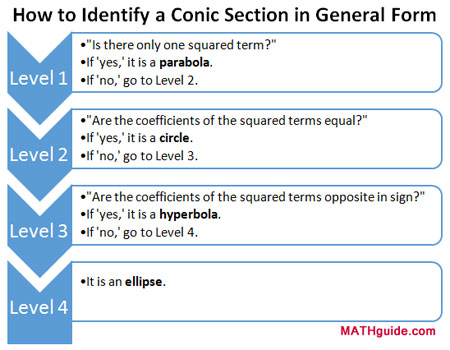It is called a process flow because the graphic informs us to start at the top and move our way down it. The steps within each level tell us what to do and all we have to do is answer a few questions.     To demonstrate how this process flow can be used, follow the example below. Example: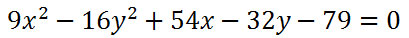We will work our way down the process flow by starting with level one. It asks, "Is there only one squared term?" Let us examine the equation to answer the question.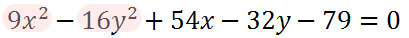Since our problem has two squared terms, we have to say "no." So, it is not a parabola and we have to go on to level two as directed.     Level two asks, "Are the coefficients of the squared terms equal?" Again, we need to look at the equation to answer.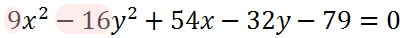After reviewing the coefficients, we have to say they are not equal. 9 is not equal to -16. So, it's not a circle and we have to proceed to level 3, as directed by the graphic.     Level three asks, "Are the coefficients of the squared terms opposite in sign?" Let's examine the graphic again.The answer is "yes." 9 is positive and -16 is negative. They do have opposite signs. They are not opposite in value, but they are opposite in that one is positive and the other is negative. According to the process flow, the conic section is a hyperbola.     It is clear that it is necessary to know basic terminology to use this process flow. Phrases like squared term, coefficient, and opposite sign need to be fully grasped to benefit by the graphic.     Test your ability to use this process flow. Make use of this quiz.uiz: Identify the Conic Section
 General Form to Standard Form The last section, called Identifying Conic Sections in General Form, demonstrated how to identify conic sections that are written in general form. This section will go one step further. It will explain how to algebraically manipulate the general form of a conic section into standard form.     In order to manipulate these equations, it is necessary to know what the equations of conics look like in standard form. View the table below to better grasp what standard form looks like.The following video will provide step by step instructions along with an example. The example targets the equation of a hyperbola.ideo: Conic Sections: General Form to Standard Formuiz: General Form to Standard Form [with help]uiz: General Form to Standard Form
 Videos Here are videos related to the lessons above.ideo: Parabolas: Directrix, Focus, Vertexideo: Parabolas: Graphingideo: Parabolas: Building Equationsideo: Graphing Ellipsesideo: Equations of Ellipses Given Foci and Verticesideo: Graphing Hyperbolasideo: Conic Sections: General Form to Standard Form
 Quizmasters After reading the lessons, try our quizmasters. MATHguide has developed numerous testing and checking programs to solidify these skills:uiz: Parabolasuiz: Equations of Circlesuiz: Graphing Ellipsesuiz: Equations of Ellipsesuiz: Graphing Hyperbolasuiz: Equations of Hyperbolasuiz: Identify the Conic Sectionuiz: General Form to Standard Form [with help]uiz: General Form to Standard Form Activities Here are activities related to the lessons above.ctivity: Equations of Circlesctivity: Ellipsesctivity: Hyperbolasctivity: Pattern Recognition for Completing the Squaresctivity: Conics: Supplementalctivity: General Form: Fill in the Blanks Related Lessons Here related lessons to view.esson: Graphing Linesesson: The Point-Slope Formulaesson: Graphing Linear Inequalitiesesson: Flight of a Projectileesson: Completing the Square